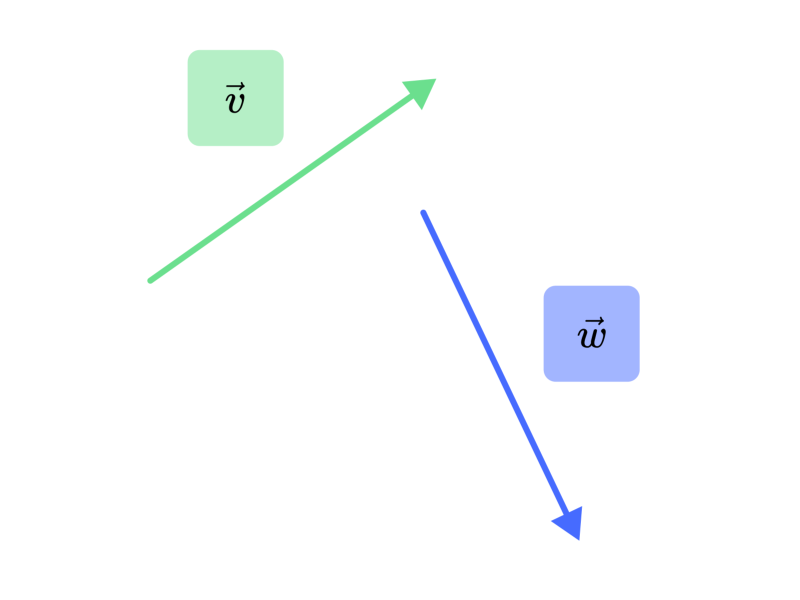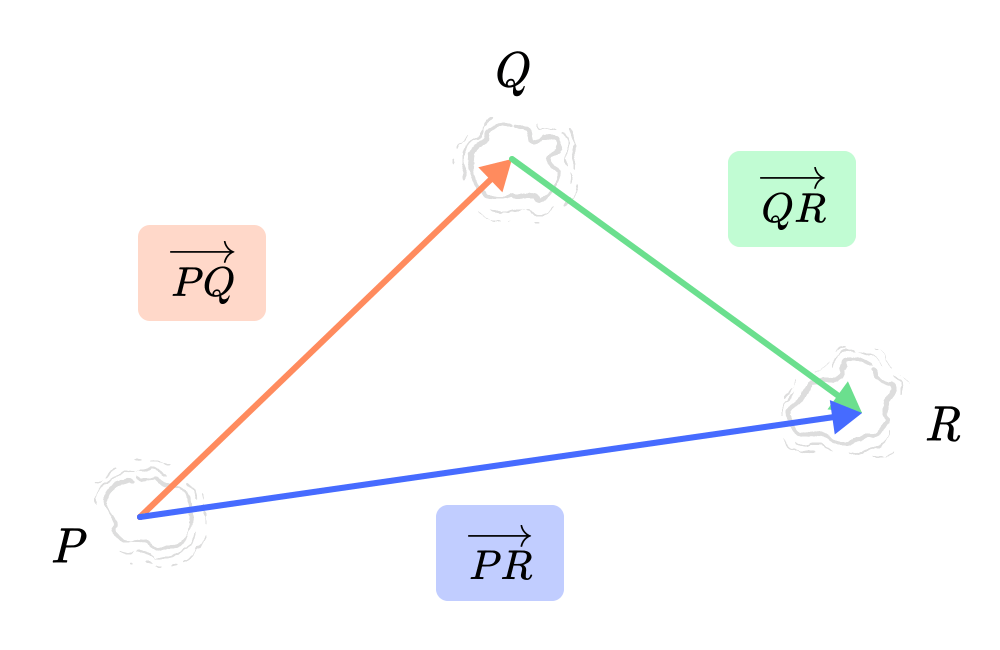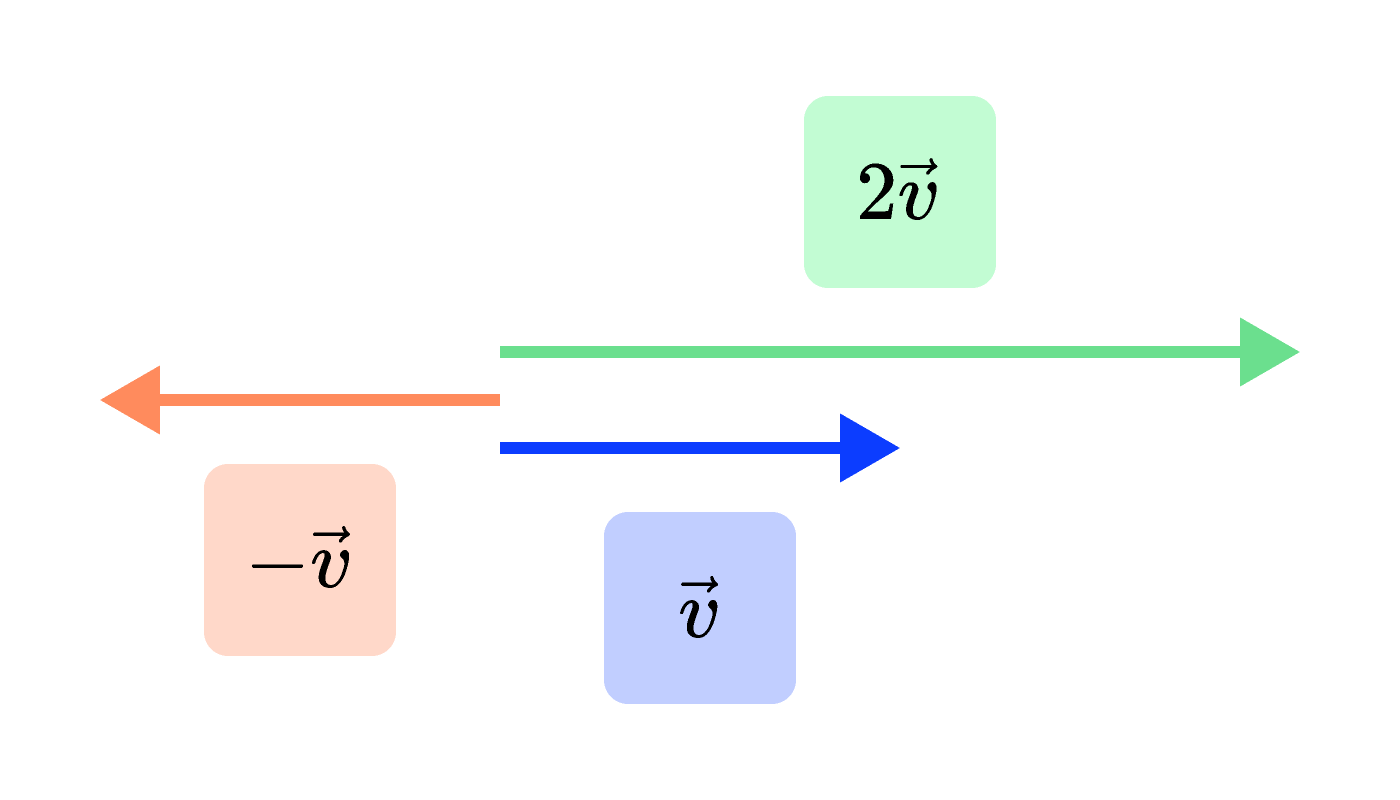### Linear Algebra with Applications

Vectors can describe any quantity with magnitude and direction.

In fact, vectors are often defined by these properties and are used to solve important problems such as the physics of motion.

But there's so much more to being a “vector,” and if we broaden our view, we can use vectors to solve a much wider range of problems.

So, what exactly is a vector then? By the end of this quiz, we'll know!

# What is a Vector?

Let's start by looking at arrows in the plane, which have both magnitude and direction.

The velocity of a bug as it walks along a tabletop is a good example. In this case, it's natural to imagine the arrow carried by the bug as it goes. Set the speed and click the play button to watch it go!

Moving arrows around the plane doesn't change their meaning at all. In fact, moving two arrows together is a natural way to add them together.

The sum $\vec{v}+\vec{w}$ is the arrow from the tail of $\vec{v}$ to the tip of $\vec{w}$ if we move $\vec{w}$ without rotating it so that its tail sits at the tip of $\vec{v}.$# What is a Vector?

For example, let's say there are three islands labeled $P,Q,$ and $R.$ A boat travels from $P$ to $Q,$ and then from $Q$ to $R.$

In other words, it first moves along $\overrightarrow{PQ}$ and then along $\overrightarrow{QR}.$

$\overrightarrow{PR},$ on the other hand, tells us where the boat starts off and where it finally stops. How is $\overrightarrow{PR}$ related to $\overrightarrow{PQ}$ and $\overrightarrow{QR} \ ?$$\overrightarrow{PQ}$ is an arrow with tail at point $P$ and head at $Q.$

# What is a Vector?

Now let's take a closer look at the sum rule.

To add $\vec{v}$ and $\vec{w}$ together, we move $\vec{w}$'s tail to $\vec{v}$'s tip; $\vec{v} + \vec{w}$ is the arrow joining $\vec{v}$'s tail to $\vec{w}$'s tip.

Do we get a different result if we instead move $\vec{v}$'s tail to $\vec{w}$'s tip?# What is a Vector?

We just saw that $\vec{v} + \vec{w}$ and $\vec{w}+\vec{v}$ are the same arrow; this means $\vec{v}+\vec{w} = \vec{w} + \vec{v}.$ So the order doesn't matter, just as when we add numbers.

But suppose we add the three arrows $\vec{u}, \vec{v},$ and $\vec{w}$ together. Does the order of addition matter now?

# What is a Vector?

To recap, arrow sums obey the "common sense" rules of commutativity $\vec{v}+\vec{w} = \vec{w} + \vec{v}$ and associativity $\vec{u} + \left( \vec{v} + \vec{w}\right) = \left( \vec{u} + \vec{v} \right) + \vec{w},$ just like real numbers.

It's hard to imagine arithmetic without these properties, so let's make them an essential aspect of "being a vector":

Vectors obey associativity and commutativity when they’re added.

# What is a Vector?

To define vectors properly, we need more than just a means of adding them together; we also need a way of multiplying them by numbers.

Take planar arrows, for instance. There's a natural way of scaling them:$2 \vec{v}$ points in the same direction as $\vec{v}$ but has twice its length; $-\vec{v} = (-1) \vec{v}$ has the same length, but points in the opposite direction.

The scalar multiplication rules for arrows are as follows:

• If $c > 0,$ then $c \vec{v}$ points in the same direction as $\vec{v}$ but has $c$ times its length.
• If $c < 0,$ then $c \vec{v}$ points in the opposite direction as $\vec{v}$ but has $|c|$ times its length.

Given what we know about arrow addition, how do we interpret $(-1)\vec{v} + \vec{v}\, ?$

# What is a Vector?

So it follows that every arrow $\vec{v}$ has an opposite $(-1)\vec{v}= - \vec{v},$ where $\vec{v} + (-\vec{v}) = \vec{0}.$ In many ways the zero arrow $\vec{0}$ plays the same role that $0$ does for real numbers.

What other properties about $\vec{0}$ and arrow scalar multiplication are true?

Select all that apply.

# What is a Vector?

Select one or more

We now extract the full definition of "vector" from all the lessons we learned studying planar arrows.

Suppose a set $V$ has the following properties:

• $V$ has addition and scalar multiplication operations that are commutative, associative, and distributive. $\\\\ \text{} \\\\$
• $V$ has a zero vector, which is an object that can be added to anything in $V$ without changing it, just like the real number 0. $\\\\ \text{} \\\\$
• Multiplying any element in $V$ by the real number $1$ doesn't change that element.

In other words, $V$ has all the same algebraic properties as planar arrows. Then we call $V$ a vector space and its elements vectors.

In the next quiz, we'll look at an example of a vector space that may just surprise you.

# What is a Vector?

×